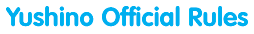## 1. The Game's Objective

Players exchange turns forming number sequences horizontally or vertically on the board, trying to score as many points as possible for each turn. The player with the highest score at the end of the game wins.

## 2. Number Placement

• One of the tiles of the first sequence must touch the center of the board.
• Every subsequent sequence must be placed so that at least one tile is shared with an existing sequence on the board.
• In a single move, tiles can only be placed in the same line vertically or horizontally.
• Tiles can be placed so that multiple new sequences are formed simultaneously using neighboring numbers.
• Sequences cannot be placed if they create an illegal sequence with their neighboring numbers.
• A sequence is legal, also called a valid "Yushino number" or "Yushino sequence," if it adheres to one of the following two rules:
1. If the sequence has 3 or more numbers, every number is the sum of the previous two numbers (modulo 10). "Modulo 10" means that when you add the two previous numbers you only look at the last digit. For example, if the previous two numbers are 6 and 8 then since 6 + 8 = 14 the next number must be 4 (we ignore the first digit, "1"). Other valid Yushino sequences are 875, since 8 + 7 = (1)5; 7291, as 7 + 2 = 9 and 2 + 9 = (1)1, etc.
2. If the sequence has 2 numbers, the numbers must differ by 1 (modulo 10). "Modulo 10" means that numbers go from 0 to 9 and then they go back to 0, so 9 is next to 0. Examples of valid two-number sequences are: 56, 87, 90, 09, 98, etc.

## 3. Scoring

• Any number placed on a square labeled "2*" or "3*" is multiplied by 2 or 3 respectively. Any "joker" numbers, i.e., blank tiles that can be turned into any number, score 0 points. The numbers on "2*" and "3*" squares for tiles that were played on previous turns are not multiplied.
• All numbers in any newly formed or modified sequence are added up, even if some were played on a previous turn.
• The total score (including any doubling or tripling of numbers as above) of any newly formed or modified sequence that touches a square labeled"2X" or "3X" is multiplied by 2 or 3 respectively. If the sequence touches two squares labeled "2X" or "3X" then it is multiplied again by 2 or 3 respectively. Sequences whose numbers were touching on "2X" or "3X" labeled squares on a previous turn do not get multiplied.
• It is possible to create multiple sequences with the same play. In this case, the score for each new sequence is computed separately, including bonuses, and the scores of all of the newly formed or modified sequences are added together.
• Sequences cannot be placed if they create an illegal sequence with their neighboring numbers.
• If a player uses all 7 of their numbers in a single turn then they are awarded a bonus 100 points.

## 4. Ending the Game

• The game ends when one player plays every tile in their rack, and there are no tiles remaining to draw from. The game also ends if three successive turns have occurred with no scoring (i.e., after three consecutive "passes").
• After the last tile is played, the opposing player will lose points equal to the sum of their remaining numbers. This amount is then awarded to the player who placed the last tile.
• If after the final calculation the players are tied in score, then the winner is the player who had the highest score before the addition/subtraction of the remaining numbers. If there is still a tie (i.e., if the tiles the opponent held are all zeroes) then the winner is Player 2, i.e., the player who placed the first move of the game loses.

## 5. Exchanging Numbers / Passing

You can exchange as many of your numbers as you want by skipping your turn. You can also just pass on your turn if you have no sequences you can place or for any other reason.
You cannot exchange your numbers in two subsequent turns - that is, after you exchange, on the next turn you have to play or you have to pass.
And you cannot exchange your numbers if there are only 7 numbers left in the central pool.

## 6. Distribution of Numbers

When the game starts, there are the following numbers in the central pool. These numbers are randomly distributed to the players as needed:
• 0: 10
• 1: 14
• 2: 14
• 3: 14
• 4: 8
• 5: 8
• 6: 8
• 7: 6
• 8: 6
• 9: 6
• Blank: 2